GFG App
Open AppBrowser
Continue

# Trigonometry

Trigonometry word is formed from the ancient Greek words “trigonon” and “metron” which mean triangle and measure respectively, thus collectively Trigonometry mean measures of a triangle. Historians believe that in ancient Greece, a mathematician named Hipparchus was the first to introduce the idea of trigonometry by giving the first tables of chords which is the modern-day equivalent to the table of values of trigonometric ratio sine. Other than Greece, roots of this subject are also found in ancient India where Aryabhatta (an Indian mathematician and astronomer) documented the modern intuition of trigonometric ratios.

## What is Trigonometry?

Trigonometry is the branch of mathematics that deals with the side and angles of a triangle, especially the right angle triangle i.e., a triangle with one angle right angle. Other than right angles, trigonometry is helpful in many different geometric figures, either 2-dimensional or 3-dimensional. Studies of Trigonometry can be classified into three parts which are as follows:

• Core Trigonometry (deal with right angle triangles only)
• Plane Trigonometry (deals with all types of 2-dimensional geometry)
• Spherical Trigonometry (deals with all types of 3-dimension geometry)

## Trigonometry Basics

Trigonometry Basics are the core concepts of trigonometry without which it can’t be defined, some of these basics of trigonometry are as follows:

• Angles: The measure of space between two intersecting lines are known as angles.
• Right-angle Triangle: A triangle with one of its interior angles being the right angle i.e., 90°, is called right angles triangle.
• Pythagoras Theorem: In right angles triangle, according to the Pythagoras theorem, the square of the hypotenuse is equal to the sum of squares of the other two sides,
• Trigonometric Ratios: Trigonometric Ratios are defined as the ratio of the sides of the right angle triangles. As there are 3 ways to choose two sides out of three and two ways for each chosen pair to arrange in ratio, thus there are 3×2 =6 trigonometric ratios which are defined for each possible pair of sides of the right angle triangle.

## Important Trigonometry Functions

The important trigonometric functions include sin and cos, as all the other trigonometric ratios can be defined in terms of sin and cos.

• tangent or tan can be defined as the ratio of sin and cos i.e., tan x = sin x/cos x,
• cotangent or cot can be defined as the ratio of cos and sin i.e., cot x = cos x/sin x, and
• cosecant or cosec is the inverse of the sin .i.e, cosec x = 1/sinx,
• secant or sec is the inverse of cos i.e., sec x = 1/cos x.

That’s why the only two important trigonometric ratios are sin and cos.

## Trigonometric Ratios

In the study of Trigonometry, there are six trigonometric ratios i.e., sin, cos, tan, cot, sec, and cosec. These trigonometric ratios as the name suggest defined as the ratios between two sides of a right-angle triangle. For the sin trigonometric ratio, we defined it as the ratio of the perpendicular (opposite to the angle for which we are calculating sin,) to the hypotenuse of the right-angle triangle i.e., for triangle ABC right angles at B,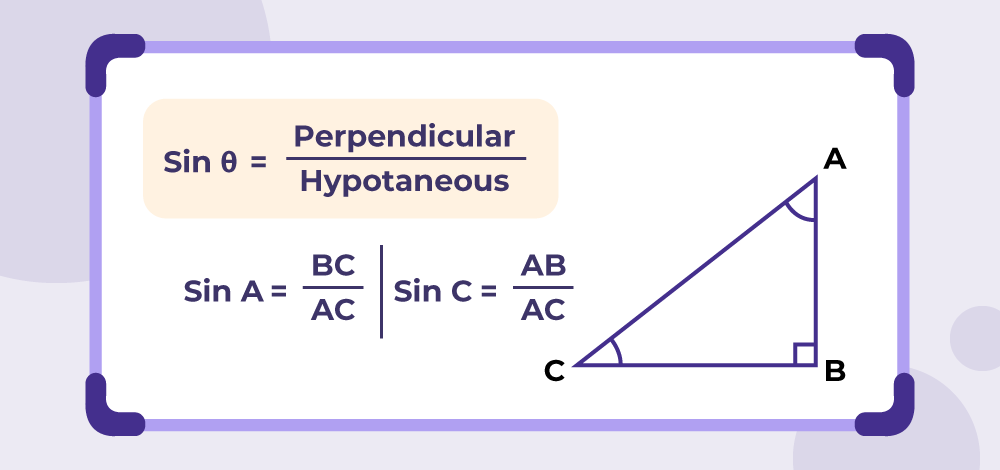Similarly, cos is defined as the ratio of the base and hypotenuse of the right-angle triangle,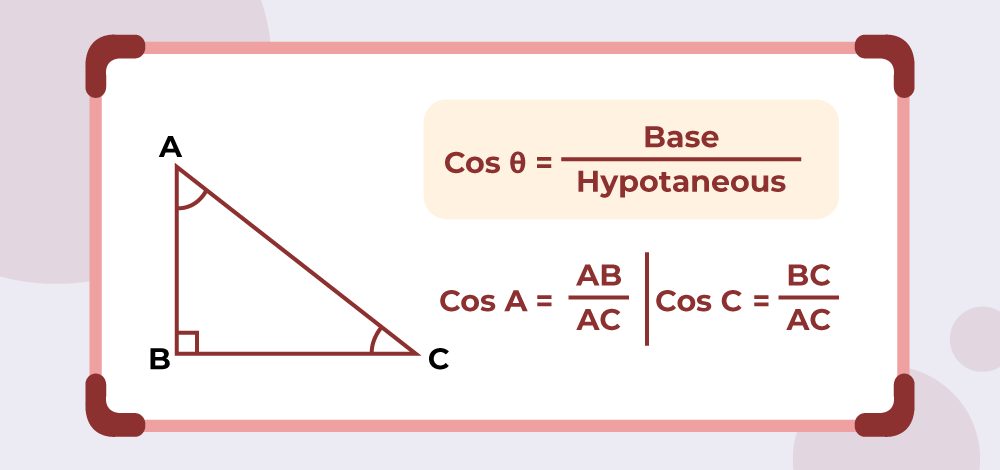and, trigonometric ratio tan is defined as the ratio of perpendicular to the base of the right-angle triangle.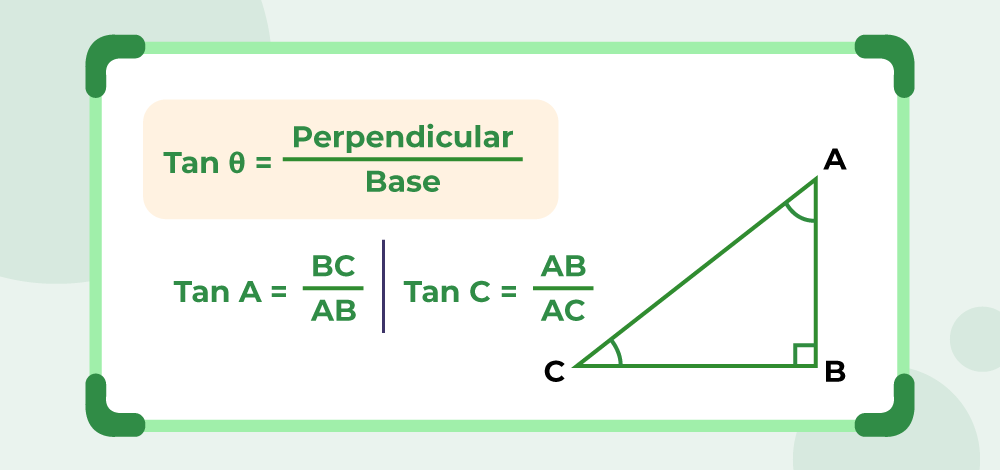Other, then sin, cos and tan, cot, sec, and cosec are also defined as the ratio of the sides of right angle triangle as follows: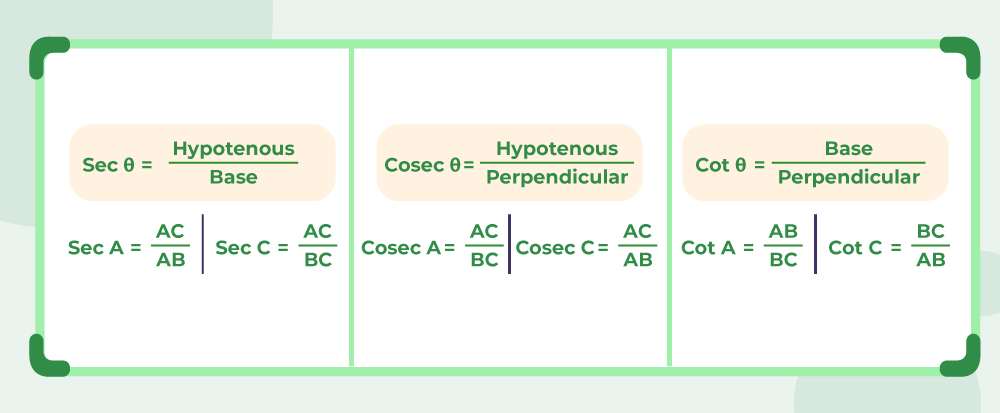Note: sec, cosec, and cot are the reciprocals of the trigonometric ratios cos, sin, and tan respectively.

## Trigonometry Table

Trigonometric Table is the table of values of trigonometric ratio at some standard angle values. The table for the value of trigonometric ratios at different angles is given below: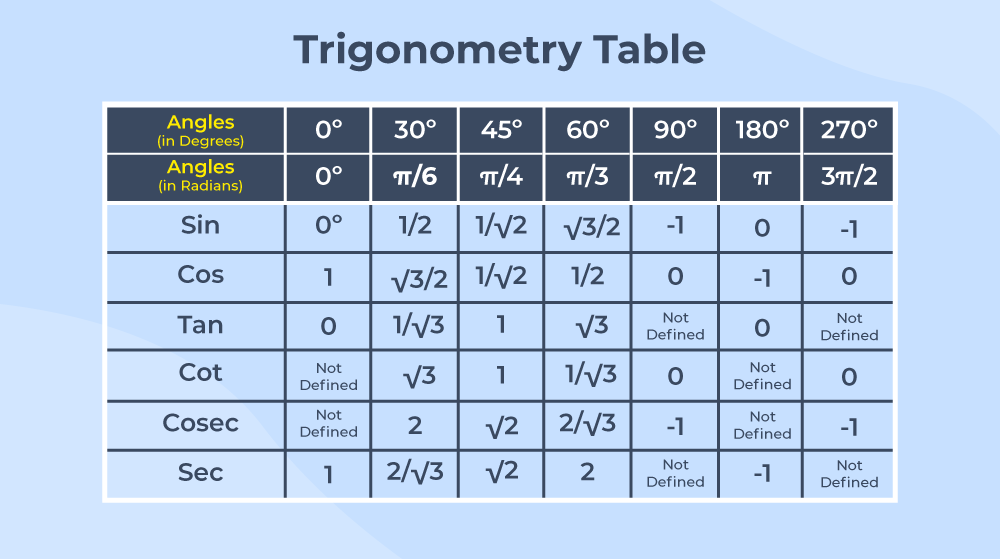## Trigonometry Chart

To create a chart of different values of trigonometric ratios at different angles, we can use the following algorithm. Using this trick we can complete the trigonometry chart without remembering the exact values of the ratios for different angles. The steps required in this algorithm are as follows:

Step 1: Write first five whole numbers with some distance between them.

0  |  1  |  2  |  3  |  4

Step 2: Divide each number by 4.

0  |  1/4  |  2/4  |  3/4  |  4/4

OR

0  |  1/4  |  1/2  |  3/4  |  1

Step 3: Take Square Root for each resulting number in step 2..

√0  |  √(1/4)  |  √(1/2)  |  √(3/4)  |  √1

OR

0  |  1/2  |  1/√2  |  √(3)/2  |  1

Step 4: Resulting values for step 3, are the value of trigonometric ratio sine for angles 0°, 30°, 45°, 60°, 90°.

Step 5: Reverse the order of the resulting values in step 3, to get the value of cos for the same angles.

1  |  √(3)/2  |  1/√2  |  1/2  |  0

Step 6: Find the ratio of the results in step 3 to results in step 5, to get the value of cos for angles 0°, 30°, 45°, 60°, 90°.

0÷1  |  1/2÷√(3)/2  |  1/√2÷1/√2  |  √(3)/2÷1/2  |  1÷0

OR

0  |  1/√3  |  1  |  √(3)  |  Not defined

Thus, using these steps, resulting table is formed.

## Trigonometry Angles

The angle for which the trigonometric ratio is defined is the trigonometric angle. Angles can either be measured in degrees (°) or can be measured in radians (rad). Some standard angles for which we create the table of trigonometric ratios are 0°, 30°, 45°, 60°, and 90°. Other than these angles, we also sometimes need to deal with 15°, 18°, 75°, and 72°.

## Application of Trigonometry

Trigonometry has so many applications in the real world, we can even say that is the most used mathematics concept throughout mathematics. Some of the applications of trigonometry are as follows:

• Trigonometry is very essential for modern-day navigation systems such as GPS or any other similar system.
• In most streams of engineering, trigonometry is used extensively for various kinds of analysis and calculations, which helps engineers to make more sound decisions for the construction of various kinds of structures.
• Various trigonometric concepts and formulas are used in the computer graphics of the modern age, as computer graphics are created in 3-D environments so all the calculations are done by the graphics processing unit of the computer to deliver the computer graphics as output.
• Various astronomical calculations such as the radius of celestial bodies, the distance between objects, etc. involve the use of trigonometry and its different trigonometric ratios.
• In Physics, we use trigonometry to understand and evaluate many real-world systems such as the orbits of planets and artificial satellites, the reflection or refraction of light ni various environments, etc.

## Trigonometry Formulas List

In trigonometry, there are a lot of formulas or identities that relate the different trigonometric ratios with each other for different values of angles. Some of these identities are:

### Pythagorean Trigonometric Identities

• sin2 θ + cos2 θ = 1
• 1+tan2 θ = sec2 θ
• cosec2 θ = 1 + cot2 θ

### Sum and Difference Identities

• sin (A+B) = sin A cos B + cos A sin B
• sin (A-B) = sin A cos B – cos A sin B
• cos (A+B) = cos A cos B – sin A sin B
• cos (A-B) = cos A cos B + sin A sin B
• tan (A+B) = (tan A + tan B)/(1 – tan A tan B)
• tan (A-B) = (tan A – tan B)/(1 + tan A tan B)

For more

Sum and Difference Identities

### Double angle Identities

• sin 2θ = 2 sinθ cosθ
• cos 2θ = cos2θ – sin 2θ = 2 cos 2 θ – 1 = 1 – sin 2 θ
• tan 2θ = (2tanθ)/(1 – tan2θ)

### Half Angle Identities

•••### Product Sum Identities

••••### Product Identities

•••### Triple Angle Identities

•••## Trigonometry Identities

An equation, which deals with different trigonometric ratios is called Trigonometric Identity if it is true for all possible values of the angles. Trigonometric identities related different trigonometric ratios i.e., sin, cos, tan, cot, sec, and cosec, with each other for various different angles. Out of all one of the most basic as well as useful identities are Pythagorean trigonometric identities which are given as follows:

sin2 θ + cos2 θ = 1

1+tan2 θ = sec2 θ

cosec2 θ = 1 + cot2 θ

## Euler’s Formula for Trigonometry

For the imaginary power of exponent e(Euler’s number), Eular gave an identity that relates the imaginary power of e to the trigonometric ratios sin and cos, the identity is given as follows:Where, i is the imaginary number which is defined as i = √(-1), and

Φ  is the angle.

Putting, instead of Φ in the above identity, we getNow, adding and subtracting these two values together we get, values of sin and cos in terms of imaginary power of Eular’s number,and## Trigonometry Examples

As Trigonometry is the most widely used concept in mathematics, it has various applications and examples in the real world. One such example is height and distance. In Height and distance, we can calculate various lengths and angles involving everyday scenarios. For example, we see a shadow of a tree on the ground and want to find the height of that tree (which is very hard to measure as we have to reach to the top of the tree to measure its height). Using trigonometry we can calculate the height of the tree without climbing the tree at all. we just need to measure the angle of the sun at the moment and the length of the shadow of the tree at the same moment and using this information we can use trigonometric ratio tan to calculate the height of the tree.## Examples on Trigonometry

### Example 1: A ladder is leaning against a wall. The angle between the ladder and the ground is 45 degrees, and the length of the ladder is 10 meters. How far is the ladder from the wall?

Solution:

Let the distance between the ladder and the wall be x meters.

Here, ladder, wall and ground together makes a right angle triangle, where for given angle,

Length of ladder = hypotenous = 10 meter,
Distance between wall and laddar = base = x meter

Using trigonometric ratio cos, we get

⇒ cos(45°) =  = x/10

⇒ cos(45°) = 1

⇒1/√2 = x/10

⇒ x = 10/√2 = 5√2 meters

Therefore, the ladder is 5√2 meters away from the wall.

### Example 2: A right-angled triangle has a hypotenuse of length 10 cm and one of its acute angles measures 30°. What are the lengths of the other two sides?

Solution:

Let’s call the side opposite to the 30° angle as ‘a’ and the side adjacent to it as ‘b’.

Now, sin (30°) = perpendicular/hypotenous = a/10

⇒ a = 10 × sin(30°) = 5 cm  [sin(30°) = 1/2]

and cos(30°) = b/10

⇒ b = 10 × cos(30°) = 10 × √(3)/2 ≈ 8.66 cm

Therefore, the lengths of the other two sides are 5 cm and 8.66 cm (approx.).

### Example 3: Prove that (cos x/sin x) + (sin x/cos x) = sec x × cosec x.

Solution:

LHS = (cos x/sin x) + (sin x/cos x)
⇒ LHS = [cos2x + sin2x]/(cos x sin x)
⇒ LHS = 1/(cosx sinx)   [Using cos2x + sin2x = 1]
⇒ LHS = (1/cosx) × (1/sinx)
⇒ LHS = secx × cosecx = RHS [ 1/cosx = sec x and 1/sinx = cosec x]

### Example 4: A person is standing at a distance of 10 meters from the base of a building. The person measures the angle of elevation to the top of the building as 60°. What is the height of the building?

Solution:

Let h be the height of the building.

and, all the distances here in the question make a right angle triangle, with a base of 10 meters and height h meter.

As tan θ = Perpendicular/Base

⇒ tan(60°) = h / 10

⇒ h = 10 tan(60°)

Using the values of tan(60°) = √3, we get:

h = 10√3 ≈ 17.32 m

Therefore, the height of the building is approximately 17.32 meters.

### Example 5: Find the value of x in the equation cos-1(x) + sin-1(x) = π/4.

Solution:

For, cos-1(x) + sin-1(x) = π/4

As we know, cos-1(x) + sin-1(x) = π/2, above equation becomes

π/2 = π/4, which is not true.

Thus, the given equation has no such value of x, which can satisfy the equation.

## FAQs on Trigonometry

### Q1: What is Trigonometry in Maths?

Trigonometry is the branch of mathematics that deals with the relationship between angles and lengths in geometric shapes.

### Q2: What are the three types of trigonometry?

The three types of trigonmetry are as follows:

• Core Trigonometry
• Plane Trigonometry
• Spherical Trigonometry

### Q3: What are the Six ratios of trigonometry?

The six trigonometric ratios are sin, cos, tan, cot, sec, and cosec.

### Q4: What is the primary function of trigonometry?

The primary function of the trigonometry is to study and understand the relationships between sides and angles of triangles.

### Q5: What are Trigonometry Identities?

An equation that holds true for all angles involving different trigonometric ratios is known as trigonometric identity.